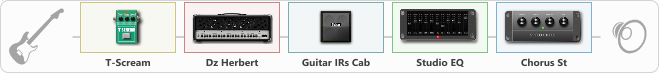# Diezel Blaster

Discussion in 'ToneLib-GFX presets' started by zainal talib, Feb 16, 2021.

1. Diezel Blaster

Preset name: Diezel @CHORUS Blaster

Effects chain:Effect: "T-Scream" (Overdrive / Distortion), active - "yes"
{
"Drive" = 35
"Tone" = 68
"Level" = 79
}

Effect: "Dz Herbert" (Amp simulators), active - "yes"
{
"Gain" = 60
"Bass" = 52
"Middle" = 45
"Treble" = 61
"Presence" = 37
"Master" = 54
"Level (dB)" = 0
}

Effect: "Guitar IRs Cab" (Cabinets), active - "yes"
{
"Model" = Fender Deluxe Rev (1x12")
"Mic Position" = Middle
"Mic Distance" = Middle
"Low Cut (Hz)" = 60
"Hi Cut (kHz)" = 20.0
"Mix" = 100
"Level (dB)" = 3
}

Effect: "Studio EQ" (Dynamics / Filter), active - "yes"
{
"31 Hz" = 0
"62 Hz" = 0
"125 Hz" = 0
"250 Hz" = 1
"500 Hz" = 2
"1 kHz" = 0
"2 kHz" = 0
"4 kHz" = 2
"8 kHz" = 0
"16 kHz" = 5
"Level (dB)" = 0
}

Effect: "Chorus St" (Modulation / Sfx), active - "yes"
{
"Speed" = 1.9
"Depth" = 31
"Center" = 4.4
"Mix" = 31
}

Note: You will need to download and install the ToneLib-GFX software to use the preset.

File size:
641 bytes
Views:
3,668